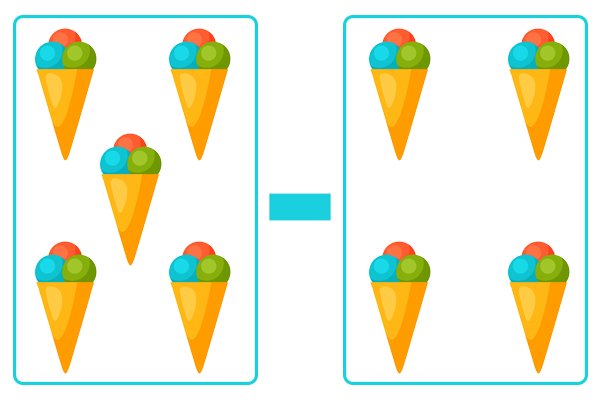# Matching Addition & Subtraction Problems

Description: Select the math problem that matches the image shown.

Question 1 (Multiple Choice, 10 points)

Select the problem below that matches this image and solve the problem.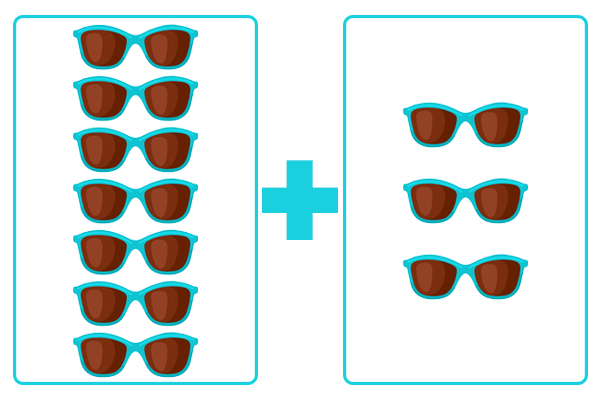Question 2 (Multiple Choice, 10 points)

Select the problem below that matches this image and solve the problem.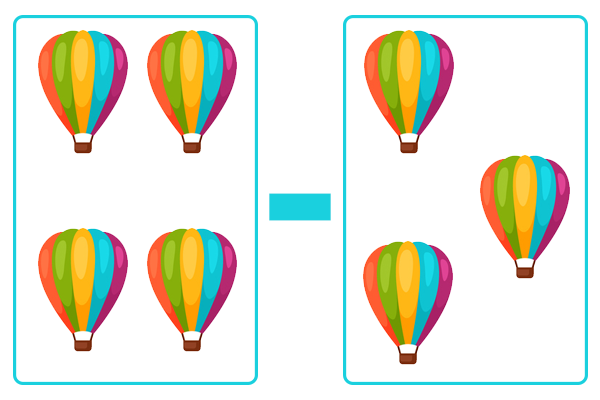Question 3 (Multiple Choice, 10 points)

Select the problem below that matches this image and solve the problem.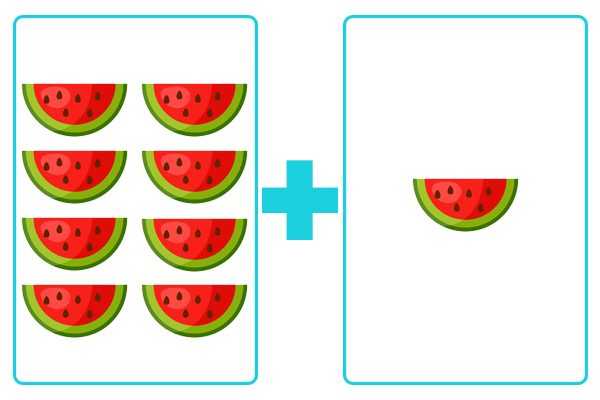Question 4 (Multiple Choice, 10 points)

Select the problem below that matches this image and solve the problem.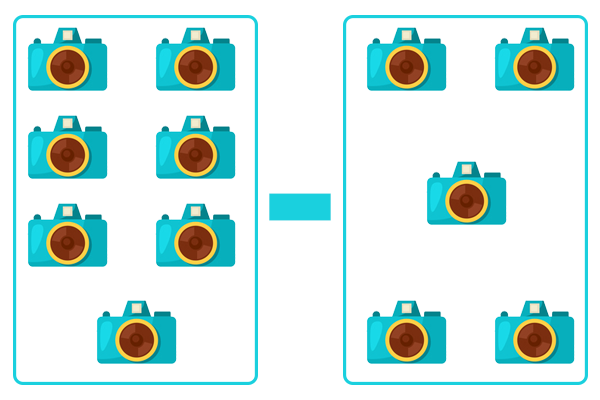Question 5 (Multiple Choice, 10 points)

Select the problem below that matches this image and solve the problem.